# A few trigonometry questions that I am confused about, Am I thinking about it wrong?

My knowledge of trigonometry on how its used in games is flucutating back and forth (I have trouble seeing the whole picture), I need to solidify it in my mind.

I have a couple questions below:
any help is appreciated.

Atan (Hypothenus) is main math function used to find angles between X and (Z or Y)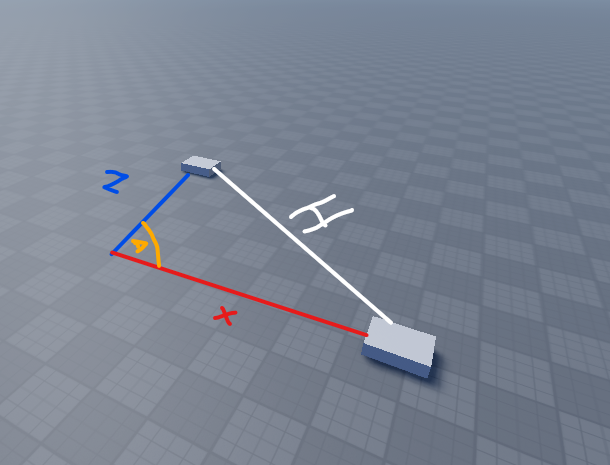Arcos(The X) is used to find angles between the X, How can it find angles purely based off the X axis?
Arcsin(The Y) is used to find angles between the Y, How can it find angles purely based off the Y axis?
They both have only one axis to measure from? so it cant be used to find angles? unless I combine it using Atan?

Can this (Atan Visual Demonstration) be used for Arcos and Arcsin to find angles between objects?Or is my definitions of Arcos, and Arcsin flawed?

2 Likes

When it comes to game development, what you really need to know is that there’s this function called Atan2 which can tell you the angle between vectors but unlike the dot product approach, it can also tell you the side it is on.

Trigonometry only works for things with right angles. The hypotenuse is always the longest side and the other sides (adjacent and opposite) are all relative to where you measure from. In your picture, for example, if we defined the angle to be between x and H then the adjacent would be x, the hypotenuse would be H and the opposite would be Z. A triangle always adds up to 180 degrees because trigonometry only works for right angles it means that the 2 angles you can calculate will add up to 90 degrees.

Sin(angle) = opposite/hypotenuse

You can apply the functions inverses (their arc functions, so atan, acos, asin) to convert the ratio to the angle which all of your ratios are relative to in order to find the angle there.

2 Likes

Am I using the formulas properly here? Acos outputs a different angle from the rest

``````local part1 = game.Workspace.Part1
local part2 = game.Workspace.part2

local opposite = (part2.Position.Z - part1.Position.Z)
local adjacent = (part2.Position.X - part1.Position.X)
local hypotenus = (part2.Position - part1.Position).Magnitude

local angleWithASin = math.asin(opposite/hypotenus)

print(math.deg(angleWithATan)) -- Output: 22
print(math.deg(angleWithASin)) -- Output: -22
print(math.deg(angleWithACOS)) -- Output: 157``````
1 Like

Even though I know absolutely nothing about trig, I’m just gonna hop in to learn something.

When you’re defining opposite and adjacent in your variables, aren’t they also supposed to have .magnitude after?

2 Likes

I just applied that and it worked, the formulas outputs the same values now, but the values dont line up.

This angle is suppose to be 90 degrees, but it ouputs to 35 degrees.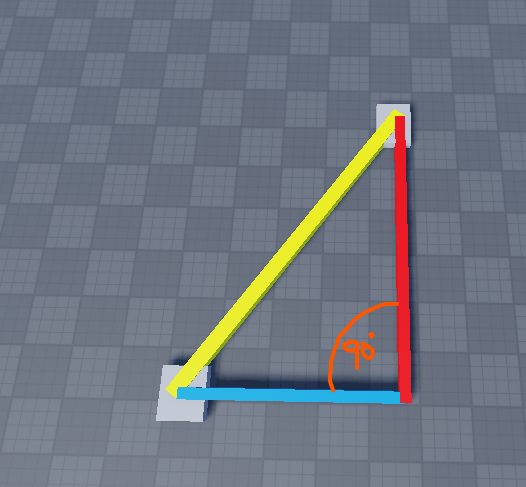Code:

``````local part1 = game.Workspace.Part1
local part2 = game.Workspace.part2

local z1 = part1.Position.Z
local z2 = part2.Position.Z
local x1 = part1.Position.X
local x2 = part2.Position.X

local opposite = (Vector3.new(0,0,z2) - Vector3.new(0,0,z1)).Magnitude
local adjacent = (Vector3.new(x2, 0,0) - Vector3.new(x1,0,0)).Magnitude
local hypotenus = (part2.Position - part1.Position).Magnitude

local angleWithASin = math.asin(opposite/hypotenus)

print(math.deg(angleWithATan)) -- Output: 35
print(math.deg(angleWithASin)) -- Output: 35
print(math.deg(angleWithACOS)) -- Output: 35

``````
1 Like

The outputs will always be the same relative to the reference of adjacent and opposite. It means that between the yellow and the blue it is 35 degrees

2 Likes

but isnt it suppose to output 90 degrees? this is a right triangle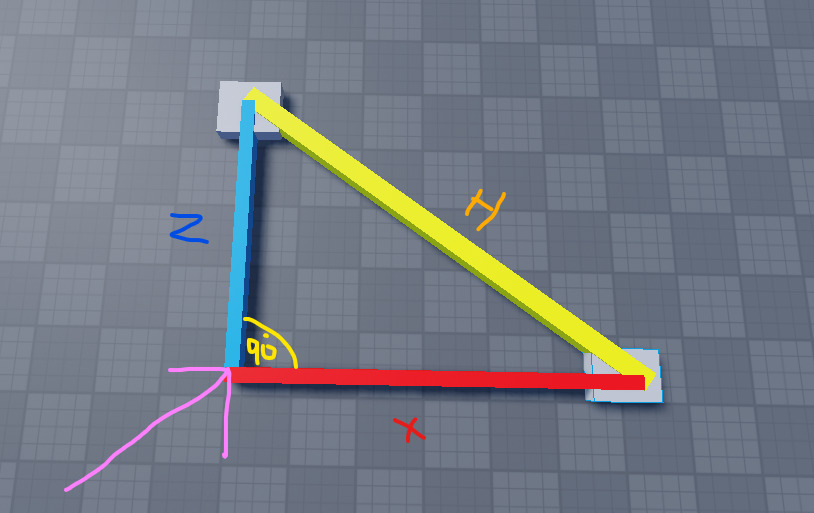1 Like

it only outputs 90 degrees when they are parralel to each other, did I do something wrong in my formula?

1 Like

It’s a bit weird because you’re talking about an angle as if it can be defined with only 2 points (the grey Parts). You always need 3 points to define an angle.

Looking at your figure you actually do have a third point, the corner of the angle, but you don’t define it as a freely moving Part like the other two points. Instead you define it as the intersection between two lines that pass through each Part and that are parallel to either the X or Z axis, respectively. This effectively locks the X and Z segments into being parallel to two different axes, and since we’re working in a right-angled coordinate system the angle between X and Z is always going to be 90 no matter where you place the points! Here’s an animation showing what I’m talking about in 2D: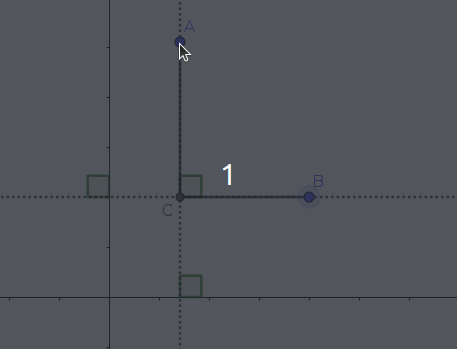As you can see, moving the points doesn’t change the angle and the line segments are always parallel to the coordinate system axes. All this means that it’s pointless to try and compute the angle like this, because the way you’ve defined the problem it’s always going to be the same independently of where the Parts are.

What you might be more interested in is the angle between the line segment formed by two Parts and some axis of the coordinate system, like this: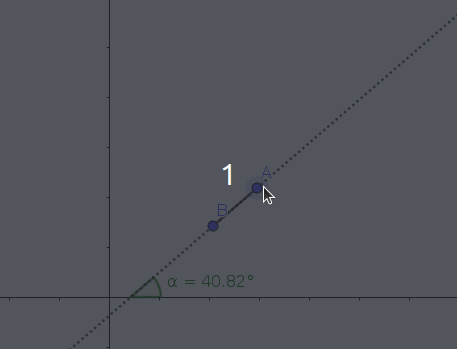It’s used for all sorts of things in games programming

1 Like

How would I do that in roblox studio?

1 Like

You technically don’t need 3 points for the triangle IF and ONLY IF it is a right triangle. You can derive the third point using the two points.

2 Likes

Is this it would look behind the scenes when calculating angles in 3d?

1 Like

I don’t understand, how would you do what?

1 Like

In one of your replies above you stated:

How do I apply the line segment between the 2 parts and compare it to one axis of the coordinate system (For example, the X axis)

1 Like

I assume I would get the magnitude distance between them? then how would I compare it to an axis, and what angles am I receiving doing that?

1 Like

I also commented on your other post, you’ve got the right technique for getting the angle between a line segment (between two parts) and the X axis.

1 Like